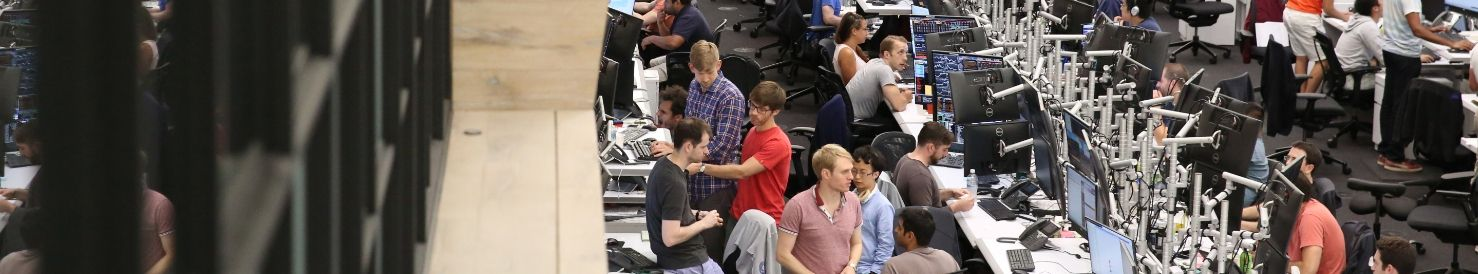Jane Street

## Interview Question

Quantitative Researcher Interview

-

# There is a solar system with three planets orbiting around the sun. One of them has a translation period of 60 years, another one of 84 years and another one of 140 years. Today, the three planets are aligned with the sun. When is the next time the three planets will be aligned with the sun?

14

Should be 210 years

1

These planets can be aligned on either the same side of the sun or opposite sides. So the answer is a number x that is the least common multiplier of 30, 42 and 70, which is 210.

Jimmy on

0

sorry i was wrong

summer zheng on

0

1/(1/60-1/84)=84*60/24=210, 1/(1/60-1/140)=140*60/80=210/2, so 210 years...

Anonymous on

0

t (in years): next time for the 3 planets align with sun t/60 - t/84 = 0.5*a t/60-t/140 = 0.5*b a and b are positive integers. Minimum t satisfied the equations above will be the answer.

ky on

0

ky's answer is right. Solve the equations you can get t=105k, where k is a positive integer. If you are not convinced, you can check that after 105 years the three planets are indeed aligned with the sun.

Fang the Spectator on

0

AB will meet every 60 years BC will meet every 210 years ABC will meet every 420 years

summer zheng on

0

any multiples of 105/2 years such as 105/2, 105, 105*3/2...

summer zheng on

3

4x3x5x7=420

Anonymous on

0

105 works too

Anonymous on